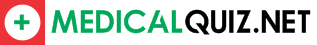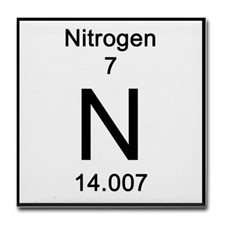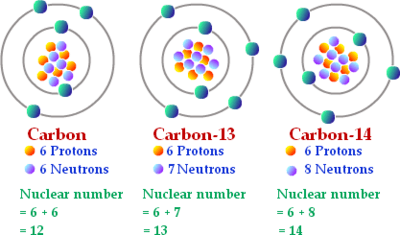Preview:What is the number of protons that the element in this image contain?
A. 14
B. 7
C. 15
D. 18

A. elements with an atomic number above 81
B. an unstable nucleus
C. contaminated sewage
D. It decays over timeAn isotope of an element has the same number of _________, but a different number of _________.

A. Protons, electrons

B. Protons, neutrons

C. Electrons, neutrons

D. Neutrons, protons

An element with a mass number of 11 and an atomic number of 5 has how many neutrons?
A. 11
B. 5
C. 6
D. 16

This particle determines what element you have – the elements identity.
A. electron
B. proton
C. neutron
D. valence shell

What particle is released when an unstable isotope undergoes alpha radiation?

A. a helium nucleus

B. an electron

C. a photon of light

D. a neutron

Where does radioactivity have application in our lives?
A. Medicine
B. Energy (electricity)
C. Agriculture
D. All of the above

Which particles make up the nucleus of an atom?
A. protons and neutrons
B. electrons and protons
C. electrons, protons, and neutrons
D. electrons and neutrons

In order of most to least penetrating radiation we have
A. Alpha , Beta, Gamma
B. Beta , Gamma , Alpha
C. Gamma, Beta, Alpha
D. Gamma, Alpha, Beta

A radioactive nuclide has a ____ stable nucleus than a non-radioactive nucleus of the same element.

A. more

B. less

C. identical

D. small

Which type of radiation releases an electron?